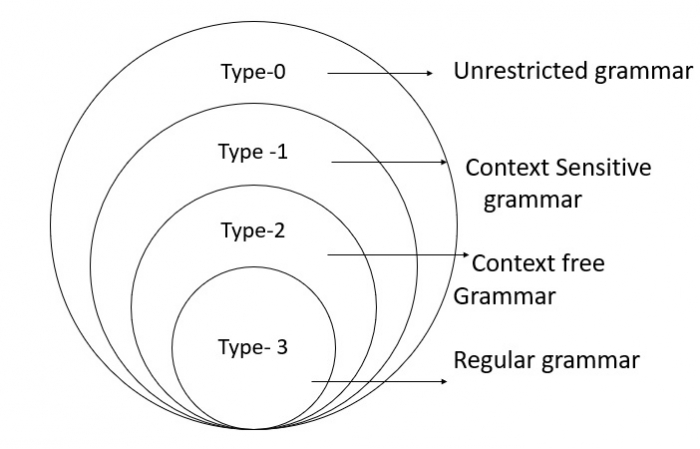# Explain Chomsky hierarchy in TOC

Chomsky Hierarchy represents the class of languages that are accepted by the different machines.

## Chomsky hierarchy

Hierarchy of grammars according to Chomsky is explained below as per the grammar types −

Type 0 − It is an Unrestricted grammars

Unrestricted grammar − an unrestricted grammar is a 4-tuple (T,N,P,S), which consisting of −

T = set of terminals

N = set of nonterminal

P =as set of productions, of the form −

v->w

where v and w are strings consisting of nonterminal and terminals.

S = is called the start symbol.

Example − Turing Machine (TM)

Type 1 − Context-sensitive grammars

All productions are of form,

v -> w where |v| < |w|

uAv -> uwv with w != epsilon,

I.e., A -> w but only in the context of u _ v.

A context-sensitive grammar is equivalent to a linear bounded and context-sensitive language.

Example  − Linear Bounded Automaton (LBA)

Type 3 − Context-free grammars −

All productions are of the form −

A -> x − where A is nonterminal, x is a string of nonterminal and terminals, A context-free grammar is equivalent to a pushdown automaton (PDA) and to context free languages.

Example − Pushdown Automaton (PDA)

Type 3 − Regular grammars

All productions are of the form −

A -> xB

A -> x where A, B are nonterminal, x is a string of terminals.

Regular grammars are equivalent to regular sets and to finite automata.

Example  − Finite Automaton (FA)

Grammar typeGrammar acceptedLanguage acceptedAutomaton
Type 0unrestricted grammarrecursively enumerable languageTuring Machine
Type 1context-sensitive grammarcontext-sensitive languagelinear-bounded automata
Type 2Context-free grammarContext-free languagePush down automata
Type 3regular grammarregular languagefinite state automaton

The Chomsky hierarchy is depicted in the diagram given below −